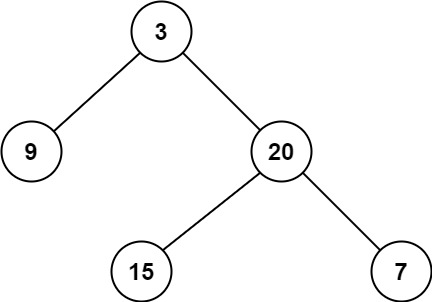# [Leetcode] 111. Minimum Depth of Binary Tree

limelimejiwon·2022년 3월 23일
0

## Python으로 공부하는 코딩테스트

목록 보기
22/67## 📄 Description

Given a binary tree, find its minimum depth.

The minimum depth is the number of nodes along the shortest path from the root node down to the nearest leaf node.

Note: A leaf is a node with no children.

### Example 1:Input: root = [3,9,20,null,null,15,7]
Output: 2

### Example 2:

Input: root = [2,null,3,null,4,null,5,null,6]
Output: 5

## 🔨 My Solution

• BFS using queue

🔴 Variables
queue: contains the nodes in the same level
minDepth: minimum depth of the tree
cur_level: saves the child nodes of the current checking node

### 🔵 Rules

• Both while roop will repeat until it reaches leaf node
• Inner while roop will repeat while queue is not empty, it means it will check the current same level of nodes
• When the inner while stops, it means there was no leaf node, so count up minDepth, and extend queue with cur_level.

## 💻 My Submission

class Solution:
def minDepth(self, root: Optional[TreeNode]) -> int:
if root is None:
return 0

# BFS using queue
queue=[root]
minDepth=1

while queue:
cur_level=[]
while queue:
node=queue.pop(0)
# if the node is a leaf node, return minDepth
if node.left is None and node.right is None:
return minDepth
else:
if node.left: cur_level.append(node.left)
if node.right: cur_level.append(node.right)
minDepth+=1
queue.extend(cur_level)

## Better BFS Solution

• save the node and level together in the queue!
• use deque and popleft()
class Solution:
def minDepth(self, root: Optional[TreeNode]) -> int:
if root is None:
return 0

# BFS using queue
queue=collections.deque([(root, 1)])

while queue:
node,level=queue.popleft()
if node:
# if the node is a leaf node, return the level
if not node.left and not node.right:
return level
else:
queue.append((node.left, level+1))
queue.append((node.right, level+1))

## Other Solution - (1) DFS

def minDepth1(self, root):
if not root:
return 0
if None in [root.left, root.right]:
return max(self.minDepth(root.left), self.minDepth(root.right)) + 1
else:
return min(self.minDepth(root.left), self.minDepth(root.right)) + 1